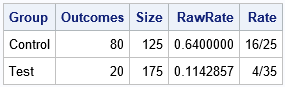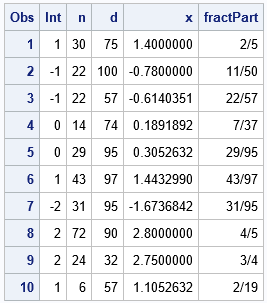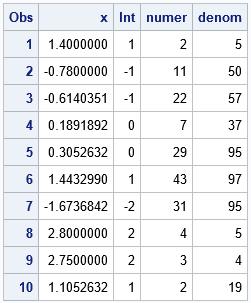I have previously shown how you can use the FRACTw. format in SAS to display numbers as fractions. But did you know that you can also use the format to obtain the numerator and denominator of the fraction as numbers in a program? All you need to do is to extract the numerator and denominator from the formatted string and convert them to integers.

### The FRACTw. format

If you are not familiar with the FRACTw. format in SAS, here is an example. Suppose a clinical trial has 125 subjects in the control group and 175 subjects in the experimental group. In the control group, 80 subjects experienced pain as compared to only 20 subjects who experienced it in the experiment group. In terms of proportions, 80/125 = 0.64 of the subjects experienced pain as compared to 20/175 = 0.1142857... in the experimental group.

Sometimes it is useful to express numbers like 0.1142857... in terms of a fraction. In this case, we know that the correct fraction is 20/175 or the reduced version of it, 4/35. However, SAS can convert decimals to fractions that are less obvious. The following example uses the FRACTw. format in SAS to display the reduced fractional form of a decimal value:

```data Trial; input Group \$ Outcomes Size; RawRate = Outcomes / Size; Rate = RawRate; /* make two copies so we can display decimal & fraction side-by-side */ datalines; Control 80 125 Test 20 175 ;   proc print data=Trial noobs; format RawRate 10.7 Rate FRACT32.; run;```In this article, I always use the FRACT32.format because 32 is the largest field width that is supported. That ensures that you can convert the widest range of decimal values to fractions.

By the way, your mathematical friends can probably convert 0.1142857... to a fraction in their head by using a trick! The trick is shown at the end of this article.

### Fractions outside of [0,1)

The FRACTw. format will represent improper fractions as a whole number plus a fraction. For example, the number 1.4 is formatted as "1+2/5". If you want to extract the whole part and the fractional parts separately, you need to find the fractional part of the number. There are two common ways to represent a number as its whole and fractional parts. For this article, I will choose to "round to negative infinity" so that the fractional part is always positive.

### Use the FRACTw. format to extract the numerator and denominator

Suppose you want to extract the numerator and denominator of the fraction. Because the numerator and denominator are integers, you can do this by extracting substrings from the formatted FRACTw. value (which is a string). Let's generate some random whole numbers and fractions to use as data:

```data Have; call streaminit(1234); do i = 1 to 10; Int = rand("Integer", -2, 2); /* integer part */ n = rand("Integer", 1, 100); /* numerator */ d = rand("Integer", 1, 100); /* denominator */ if n>d then do; tmp=n; n=d; d=tmp; end; /* swap so that n<=d */ fractPart = n / d; /* decimal version of n/d */ x = Int + fractPart; output; end; format x 10.7 fractPart FRACT32.; keep Int n d x fractPart; run;   proc print data=Have; var Int n d x fractPart; run;```Notice that the X value contains some values that are easy to convert to fractions (for example, 1.4, 2.75) but other values (1.4432990) whose fractional form (43/97) is less obvious. The goal is to use SAS to represent the X values as a whole number plus a fraction in reduced form without peeking at the N and D variables. As you can see from the previous table, the FRACT32. format performs the work for you. You can use the following steps to extract the numerator and denominator into numerical variables:

• Decompose the number into its integer and fractional parts.
• For the fractional part, use the PUT statement and the FRACTw. format to create a string of the form "NUMER/DENOM".
• Find the position of the '/' character. The substring to the left contains the numerator; the substring to the right contains the denominator.
• Use the INPUT function and the w.d informat to extract numerical values from the substrings.

The following DATA step carries out the method to represent each decimal value as an integer and a fraction. The Numer and Denom variables contain the numerator and denominator, respectively, of the fractional part of X.

```data NumerDenom; set Have(keep=x); /* read in x, the data to convert */ /* x = floor(x) + frac(x) where frac(x) >= 0 https://blogs.sas.com/content/iml/2020/02/10/fractional-part-of-a-number-sas.html */ Int = floor(x); /* integer part of x */ f = x - Int; /* fractional part, f, is always in [0,1) */ s = put(f, FRACT32.); /* convert to fraction as a string */ slashPos = find(s,'/'); /* find the '/' character */ if slashPos=0 then do; /* if value can't be represented as fraction */ numer = input(s, 32.); denom = 1; /* for example: 0 = 0/1 */ end; else do; numer_str = substr(s, 1, slashPos-1); /* get string to the left of '/' */ denom_str = substr(s, slashPos+1); /* get string to the right of '/' */ numer = input(numer_str, 32.); /* convert numerator string to integer */ denom = input(denom_str, 32.); /* convert denominator string to integer */ end; drop f s slashPos numer_str denom_str; run;   proc print data=NumerDenom; run;```Success! The method correctly converted decimal values into an integer part and a fraction. The numerator and denominator of the fraction are stored in separate numerical variables.

### Summary

SAS contains many formats that convert numbers into strings. One interesting format is the FRACTw. format, which enables you to display a decimal value as an integer and a fraction. If you want to extract the numerator and denominator of the fraction, you can extract the numerator and denominator from substrings of the formatted value. You can then use the INPUT function to convert the strings to numeric values.

### Appendix: Mental calculation of 0.1142857...

My mathematical readers might scoff, "We don't need SAS to know that 0.1142857... equals 4/35! We can do that calculation in our heads!" The trick is recognizing that the numeric sequence "142857" is part of the repeating decimal expansion of the fraction 1/7 = 0.142857.... If you know that fact, then you can split the decimal 0.1142857... into two parts: 0.1 + 0.0142857.... This enables you to recognize the decimal as 1/10 + (1/7)/10 = 8/70, which you can easily reduce to 4/35 in your head.

The post Convert decimals to fractions in SAS appeared first on The DO Loop.